# DBERlibR-vignette

This is a documentation of DBERlibR, which represents Discipline-based Education Research library R. The package runs R scripts to clean the data, merge/bind multiple data sets (as necessary), check assumption(s) for a specific statistical technique (as necessary), and run the main assessment data analysis all at once. The output(s) contain(s) a sample interpretation of the results for the convenience of users. Users need to prepare the data file as instructed and type a function in the R console (with the data file name(s)) to conduct a specific data analysis.

## Load DBERlibR, Set Working Directory, and Save Data File(s) in that Directory

Load DBERlibR by typing/copying the line below in the R console and hitting the Enter key.

library(DBERlibR)

Then, users need to set “Working Directory” under the “Session” menu in R Studio. The directory should be a folder where (all) data file(s) is(are) saved because the functions in this package import accurately named data file(s) from the working directory. Sample data files for users to test the functions and view examples have been downloaded into users’ computer when installing DBERlibR; run the ‘system.file(“extdata”, package = “DBERlibR”)’ in the console panel, and users will see the path to the downloaded data folder (i.e., extdata).

system.file("extdata", package = "DBERlibR")
#>  "C:/Users/Minjae Song/AppData/Local/Temp/RtmpMjAnvB/Rinst154452dd4c34/DBERlibR/extdata"

## Data Preparation

The data should include only (student) id and questions (no other data like demographic information; users should save other data in a separate file along with id if users have them). The question data should be binary (i.e., 1 for correct answers, 0 for incorrect answers). The data file should look like the sample below. The data file should be in the “csv” format.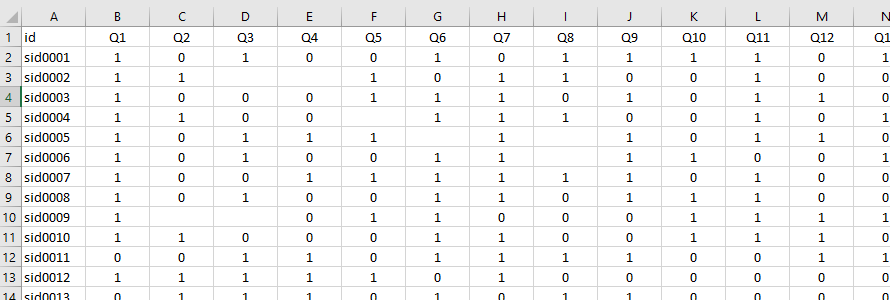Data File Format

As users can see in the data table above, the questions were named Q1, Q2, Q3, and so on, but users can name the questions differently; make sure to use the same question (column in the csv file) names across different data sets (e.g., treatment group pre-test data, treatment group post-test data, control group pre-test data, and control group post-test data).

Skipped answers in the data file(s) (i.e., blank cells in the data frame above) will be treated as incorrect in this package. Too many skipped answers may skew the results of data analysis. Users can exclude students with too many skipped answers by defining too many skipped answers; users can just provide a percentage as an argument (m_cutoff) in the function.

If users have students’ demographic information and want to examine the differences of demographic subgroups (e.g., male vs. female), they should save those demographic data in a separate file along with id (the same id as in the assessment data file(s)). The data format should look like the one below. DO NOT convert the subgroup label to a numeric code; it will be hard to read the results if users use a numeric code.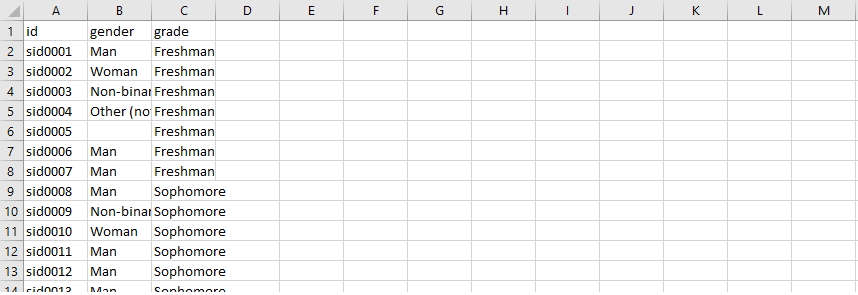Demographic Data File Format

## Item Analysis Function: “item_analysis(score_csv_data, m_cutoff = 0.15)”

The item analysis function requires users to type a data file name as shown in the sample code below. Users can input any of the data file names (e.g., “data_treat_pre.csv”, “data_treat_post.csv”, “data_ctrl_pre.csv”, or “data_ctrl_post.csv”) as they become available for item analysis.

An example without a file path: item_analysis(score_csv_data = “data_treat_pre.csv”, m_cutoff = 0.1). If you provide only data file name, as shown in the function above, the folder where the file is saved should be set as the working directory. Alternatively, you can provide the path to the folder in the argument.

Alternative example with a file path: item_analysis(score_csv_data = “C:/Users/csong/documents/data_treat_pre.csv”, m_cutoff = 0.1). The ‘m_cutoff = 0.1’ in the function indicates removing students with 10 percent or more skipped answers (the default is 15 percent: m_cutoff = 0.15).

The function will automatically reads and cleans the data (e.g., deleting students with too many skipped answers, converting missing values to “0”), calculates difficulty and discriminant scores, and displays all results in the Console and Plots panels of RStudio.

Item difficulty refers to the proportion of students that correctly answered the item, scaled 0 to 1. Ideal values are known to be slightly higher than midway between a chance to be chosen (i.e., 1 divided by the number of choices) and a perfect score (1) for the item. In common, values below 0.2 are considered to indicate “very difficult” items and need to be reviewed for possible confusing language, removed from the test, and/or identified as an area for re-instruction to improve test instruments. In addition to improving the test instrument, the results of item difficulty analysis inform the areas of weakness in students’ knowledge to be taken into consideration in improving teaching modules. The difficulty plot is shown below.

#> Item Analysis Results - Difficulty
#>     q.number difficulty_index
#> 1         Q1             0.86
#> 2         Q2             0.44
#> 3         Q3             0.70
#> 4         Q4             0.36
#> 5         Q5             0.46
#> 6         Q6             0.62
#> 7         Q7             0.60
#> 8         Q8             0.48
#> 9         Q9             0.64
#> 10       Q10             0.58
#> 11       Q11             0.70
#> 12       Q12             0.60
#> 13       Q13             0.36
#> 14       Q14             0.54
#> 15       Q15             0.78
#> 16       Q16             0.72
#> 17       Q17             0.64
#> 18       Q18             0.38
#> 19       Q19             0.58
#> 20       Q20             0.56
#> 21       Q21             0.38
#> 22       Q22             0.52
#> 23 avg_score             0.57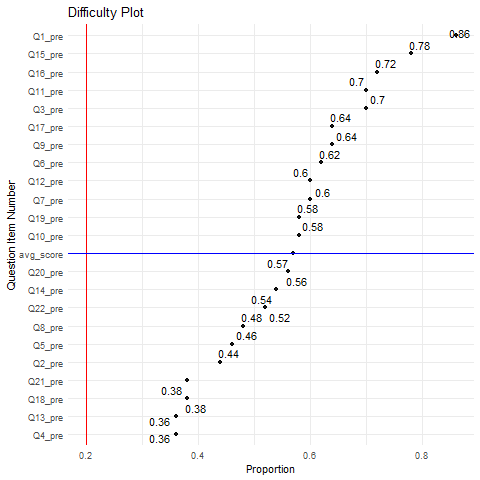Difficulty Plot

#> Refer to 'Difficulty Plot' in the 'Plots' panel.
#> As seen in the difficulty plot, none of the difficulty indixes was found to be lower than 0.2.

The proportions in the generated difficulty plot above are ordered by their size, and the plot displays a vertical red-colored line that represents the threshold of 0.2 so that users can instantly find the question items that warrant users’ attention for improvement. The plot above does not have any item of which proportion size is below 0.2.

Meanwhile, item discrimination represents the relationship between how well students did on the item and their total test performance, ranging from 0 to 1. The closer to 1, the better; the scales of 0.2 or higher are generally known to be acceptable. Items with discrimination values near or less than zero need to be removed from the test. For example, negative values indicate that students who did poorly on the test did better on that item than those who overall did well, which doesn’t make sense. Removing the items highlighted in red, especially with negative values, in the table may need to be considered, or improving instructions related to the items with low values should be considered to increase the values. The discrimination plot is shown below.

#> Item Analysis Results - Discrimination:
#>      qnumber discrimination_index
#> 1         Q1                -0.12
#> 2         Q2                -0.25
#> 3         Q3                 0.15
#> 4         Q4                 0.08
#> 5         Q5                -0.16
#> 6         Q6                 0.09
#> 7         Q7                 0.08
#> 8         Q8                 0.19
#> 9         Q9                 0.33
#> 10       Q10                 0.14
#> 11       Q11                 0.30
#> 12       Q12                 0.29
#> 13       Q13                 0.53
#> 14       Q14                 0.16
#> 15       Q15                 0.17
#> 16       Q16                 0.48
#> 17       Q17                -0.06
#> 18       Q18                 0.57
#> 19       Q19                 0.31
#> 20       Q20                 0.55
#> 21       Q21                 0.30
#> 22       Q22                 0.28
#> 23 avg_score                 1.00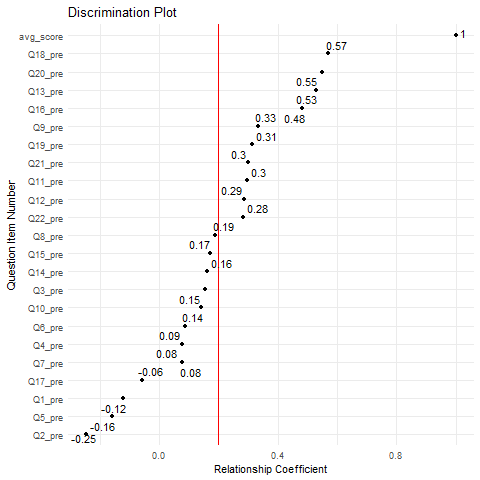Discrimination Plot

#> Refer to 'Discrimination Plot' in the 'Plots' panel
#> As seen in the discrimination plot, the following question items present a discrimination index lower than 0.2:
#>   "Q1"  "Q2"  "Q3"  "Q4"  "Q5"  "Q6"  "Q7"  "Q8"  "Q10" "Q14" "Q15" "Q17"

The relationship coefficients in the generated discrimination plot are ordered by their size, and the plot displays a vertical red-colored line that represents the threshold of 0.2 so that users can instantly find the question items that warrant users’ attention for improvement. The plot above presents 12 question items of which coefficients are less than 0.2.

## Paired Samples Data Analysis Function: “paired_samples(pre_csv_data, post_csv_data, m_cutoff = 0.15)”

An ideal situation for examining the effect of teaching modules on student performance (i.e., pre-post difference) is to have a control group to compare with the treatment/intervention group. However, it is often difficult to get the control group data. If that is the case, users can use this function to examine the difference between pre-test and post-test scores of the treatment group.

An example without a file path: paired_samples(pre_csv_data = “data_treat_pre.csv”, post_csv_data = “data_treat_post.csv”, m_cutoff = 0.1). If you provide only data file names, as shown in the function above, the folder where the file is saved should be set as the working directory. Alternatively, you can provide the path to the folder in the argument.

Alternative example with a file path: paired_samples(pre_csv_data = “C:/Users/csong/documents/data_treat_pre.csv”, post_csv_data = “C:/Users/csong/documents/data_treat_post.csv”, m_cutoff = 0.1). The ‘m_cutoff = 0.1’ in the function indicates removing students with 10 percent or more skipped answers (the default is 15 percent).

The function automatically cleans the data sets (e.g., converting missing values to “0), merges pre-post data sets, check assumptions, and then runs the Paired Samples T-test (Parametric) and Wilcoxon Signed-Rank test (Nonparametric) to help users examine the difference between pre-post scores. The outputs of the function are as follows.

First, a summary of pre-/post-test scores’ descriptive statistics will be presented in the R console.

#> # A tibble: 2 × 11
#>   Time  variable     n   min   max median   iqr  mean    sd    se    ci
#>   <fct> <fct>    <dbl> <dbl> <dbl>  <dbl> <dbl> <dbl> <dbl> <dbl> <dbl>
#> 1 Pre   Score       45 0.364 0.773  0.545 0.136 0.572 0.097 0.014 0.029
#> 2 Post  Score       45 0.364 0.864  0.636 0.136 0.655 0.111 0.017 0.033

Then, boxplots will be presented in the ‘Plots’ panel in RStudio to help users visually inspect the descriptive statistics.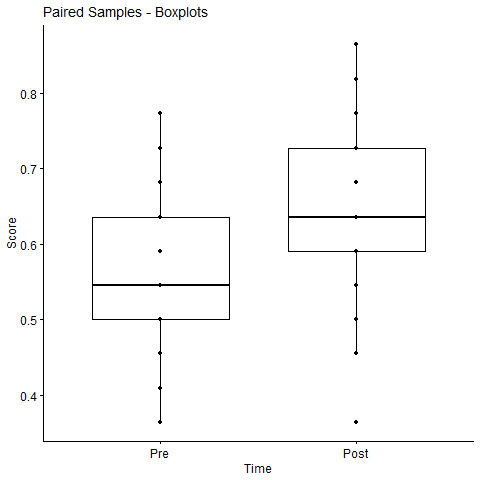Paired Samples - Boxplots

Second, the function runs scripts to check the assumptions (no outliers and normal distribution of of mean differences) to be satisfied for using the paired samples t-test and present the results in the R console. For example, users can see the result of the Shapiro-Wilk normality test in the R console (followed by a brief interpretation of the result: e.g., “the assumption of normality by group has NOT been met (p<0.05)) and the histogram and normal Q-Q plot of the mean differences, as shown below.

#> # A tibble: 1 × 3
#>   variable statistic      p
#>   <chr>        <dbl>  <dbl>
#> 1 avg_diff     0.952 0.0607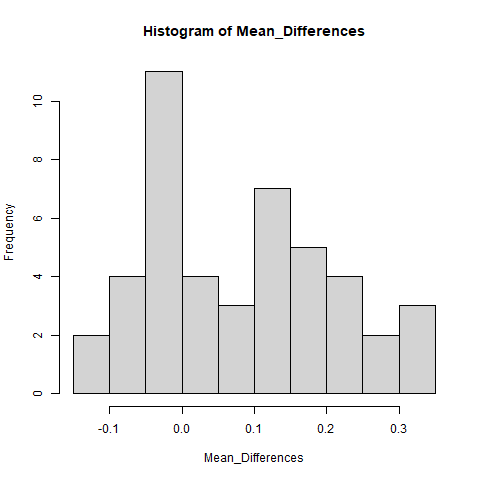Paired Samples - Histogram

If the sample size is greater than 50, it would be better refer to the QQ plots displayed in the ‘Plots’ panel to visually inspect the normality. This is because the Shapiro-Wilk test becomes very sensitive to a minor deviation from normality at a larger sample size.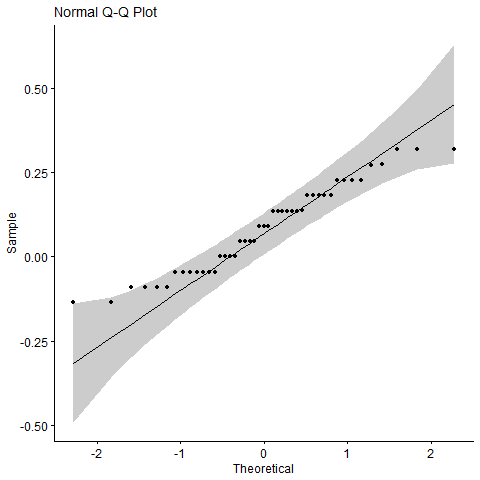Paired Samples - Normal Q-Q Plot

Third, the main paired samples t-test result is presented in the R console, followed by a brief interpretation of the result: e.g., “The average pre-test score was 0.57 and the average post-test score was 0.66. The Paired Samples T-Test showed that the pre-post difference was statistically significant (p<0.001)”, as shown below.

#>
#>  Paired t-test
#>
#> data:  treat_data_merged$avg_score_pre and treat_data_merged$avg_score_post
#> t = -4.2903, df = 44, p-value = 9.625e-05
#> alternative hypothesis: true mean difference is not equal to 0
#> 95 percent confidence interval:
#>  -0.12319734 -0.04444711
#> sample estimates:
#> mean difference
#>     -0.08382222

Fourth, if the sample size is considered to be too small (e.g., less than 15), or the data has failed to satisfy the normality assumption for using the parametric paired samples t-test, then the function automatically runs the Wilcoxon signed rank sum test (a non-parametric version of the paired samples t-test) and presents its results in the R console, as shown below. Users won’t be able to see the non-parametric test result if they can use the parametric test result, since the parametric test is more powerful than its non-parametric alternative.

#>
#>  Wilcoxon signed rank test with continuity correction
#>
#> data:  treat_data_merged$avg_score_pre and treat_data_merged$avg_score_post
#> V = 146.5, p-value = 0.0002332
#> alternative hypothesis: true location shift is not equal to 0

Finally, the function further runs scripts to run McNemar test to examine the difference between pre-post scores for individual test items. The information generated from this function is useful in determining which items would have been signficantly impacted by the treatment/intervention (e.g., teaching modules). As shown in the plot below, the function generates a plot to show the size of p-values (significance of the pre-post difference) for individual test items (ordered by the p-values). The plot displays a vertical line to show a threshold of statistical significance (p=0.05), so that users can easily find test question items of which the difference between pre-post scores is statistically significant. A brief summary of the plot is provided in the R console for the convenience of users: e.g., “the plot shows the statistical significance of the difference between pre-post scores of the test items”Q4,” “Q7,” and “Q17.”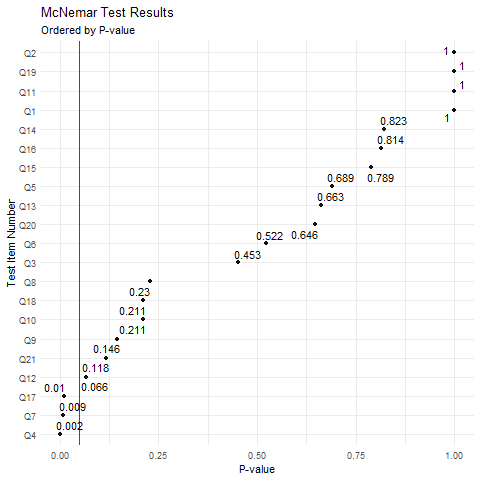Paired Samples - Mean Differences - Individual Items

## Indepent Samples Data Analysis Function: “independent_samples(treat_csv_data, ctrl_csv_data, m_cutoff = 0.15)”

Ideally, it requires both pre-test and post-test data to examine the difference between the treatment and the control group. However, it is often difficult to get the pre-test data. If that is the case, users can use this function to examine the difference between two (treatment vs. control) groups. Please make sure to name data files accurately (i.e., “data_treat_post.csv” and “data_ctrl_post.csv”) and have them saved in the working directory.

An example without a file path: independent_samples(treat_csv_data = “data_treat_post.csv”, ctrl_csv_data = “data_ctrl_post.csv”, m_cutoff = 0.1). If you provide only data file names, as shown in the function above, the folder where the file is saved should be set as the working directory. Alternatively, you can provide the path to the folder in the argument independent_samples

Alternative example with a file path: independent_samples(treat_csv_data = “C:/Users/csong/documents/data_treat_post.csv”, ctrl_csv_data = “C:/Users/csong/documents/data_ctrl_post.csv”, m_cutoff = 0.1). The ‘m_cutoff = 0.1’ in the function indicates removing students with 10 percent or more skipped answers (the default is 15 percent; m_cutoff = 0.15).

This function automatically cleans the data sets (e.g., converting missing values to “0), binds treatment-control group data sets, check assumptions, and then runs the Independent Samples T-test (parametric) and Mann–Whitney U test (nonparametric) to help users examine the difference between the groups. The outputs from this function are as follows.

First, a summary of treatment/control groups’ descriptive statistics will be presented in the R console.

#> # A tibble: 2 × 5
#>   datagroup variable           n  mean    sd
#>   <fct>     <fct>          <dbl> <dbl> <dbl>
#> 1 Control   avg_score_post    54 0.588 0.107
#> 2 Treatment avg_score_post    45 0.655 0.111

Then, boxplots will be presented in the ‘Plots’ panel in RStudio to help users visually inspect the descriptive statistics.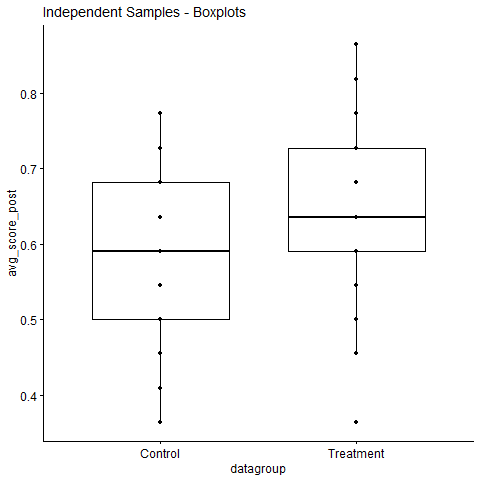Independent Samples - Boxplots

Second, the function run scripts to check the assumptions (no outliers, normality for each gruop) to be satisfied for using the independent samples t-test (parametric) and present the results in the R console. Users will have the result of the Shapiro-Wilk normality test in the R console (followed by a brief interpretation of the result: e.g., “Interpretation: the assumption of normality has been met (p>0.05 for each group)), as shown below.

#> # A tibble: 2 × 4
#>   datagroup variable       statistic     p
#>   <fct>     <chr>              <dbl> <dbl>
#> 1 Control   avg_score_post     0.965 0.118
#> 2 Treatment avg_score_post     0.970 0.288
#> ## Interpretation: the assumption of equality of variances has been met (p>0.05)

If the sample size is greater than 50, it would be better refer to the normal Q-Q plot displayed in the ‘Plots’ panel to visually inspect the normality. This is because the Shapiro-Wilk test becomes very sensitive to a minor deviation from normality at a larger sample size.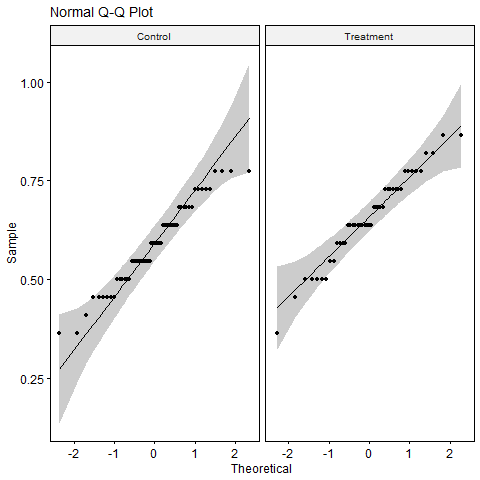Independent Samples - Normal Q-Q Plots

Then, the function proceeds to check the assumption of equal variances between two independent groups and shows the test results with an interpretation of the result, as shown below.

#> # A tibble: 1 × 4
#>     df1   df2 statistic     p
#>   <int> <int>     <dbl> <dbl>
#> 1     1    97    0.0344 0.853
#> ## Interpretation: the assumption of equality of variances has been met (p>0.05)

Third, the main independent samples t-test result is presented in the R console, followed by a brief interpretation of the result: “The treatment group’s average score was 0.66, and the control group’s average score was 0.59. The Independent Samples T-Test showed that the group difference was statistically significant (p=0.003).”, as shown below. The function presents the results with equal variances assumed or not assumed, based on the test result of equal variances between two groups.

#>
#>  Welch Two Sample t-test
#>
#> data:  group_data_binded$avg_score_post by group_data_binded$datagroup
#> t = -3.0493, df = 92.636, p-value = 0.002991
#> alternative hypothesis: true difference in means between group Control and group Treatment is not equal to 0
#> 95 percent confidence interval:
#>  -0.11089206 -0.02341905
#> sample estimates:
#>   mean in group Control mean in group Treatment
#>               0.5883333               0.6554889

Finally, if either the sample size is too small (e.g., less than 15) or the data fails to satisfy the normality assumption, then the function further runs scripts for the Mann-Whitney U test (a non-parametric version of the independent samples t-test) and presents its results in the R console, as shown below (Note: users won’t be able to see the non-parametric test result if the data satisfies the normality assumption since the parametric test is more powerful than its non-parametric alternative).

#>
#>  Wilcoxon rank sum test with continuity correction
#>
#> data:  group_data_binded$avg_score_post by group_data_binded$datagroup
#> W = 807.5, p-value = 0.003946
#> alternative hypothesis: true location shift is not equal to 0

## One-way ANCOVA Function: “one_way_ancova(treat_pre_csv_data, treat_post_csv_data, ctrl_pre_csv_data, ctrl_post_csv_data, m_cutoff)”

The function “one_way_ancova()” is to conduct a one-way analysis of covariance (ANCOVA). The ANCOVA is an extension of the one-way ANOVA to incorporate a covariate variable into the analytic model. The inclusion of this covariate (pre-test scores in this function), which is linearly related to the dependent variable (e.g., post-test scores), into the analysis increases the ability to detect differences between groups of an independent variable (treatment vs. control group in this function).

An example without a file path: one_way_ancova(treat_pre_csv_data = “data_treat_pre.csv”, treat_post_csv_data = “data_treat_post.csv”, package = “DBERlibR”), ctrl_pre_csv_data = “data_ctrl_pre.csv”, ctrl_post_csv_data = “data_ctrl_post.csv”, m_cutoff = 0.1). If you provide only data file names, as shown in the function above, the folder where the file is saved should be set as the working directory. Alternatively, you can provide the path to the folder in the argument independent_samples

Alternative example with a file path: one_way_ancova(treat_pre_csv_data = “C:/Users/csong/documents/data_treat_pre.csv”, treat_post_csv_data = “C:/Users/csong/documents/data_treat_post.csv”, ctrl_pre_csv_data = “C:/Users/csong/documents/data_ctrl_pre.csv”, ctrl_post_csv_data = “C:/Users/csong/documents/data_ctrl_post.csv”, m_cutoff = 0.1)). The ‘m_cutoff = 0.1’ in the function indicates removing students with 10 percent or more skipped answers (the default is 15 percent; m_cutoff = 0.15).

This function automatically merges pre-post data sets, binds treatment-control data sets, runs scripts to check assumptions of one-way ANCOVA, and then runs the main One-way ANCOVA all at once. The outputs from this function are as follows. The outputs/results from this function are as follows.

First, the function presents descriptive statistics and boxplots of all four groups, as shown below.

#> # A tibble: 2 × 5
#>   datagroup  mean     sd   min   max
#>   <fct>     <dbl>  <dbl> <dbl> <dbl>
#> 1 Control   0.567 0.100  0.318 0.727
#> 2 Treatment 0.572 0.0971 0.364 0.773
#> # A tibble: 2 × 5
#>   datagroup  mean    sd   min   max
#>   <fct>     <dbl> <dbl> <dbl> <dbl>
#> 1 Control   0.592 0.107 0.364 0.773
#> 2 Treatment 0.655 0.111 0.364 0.864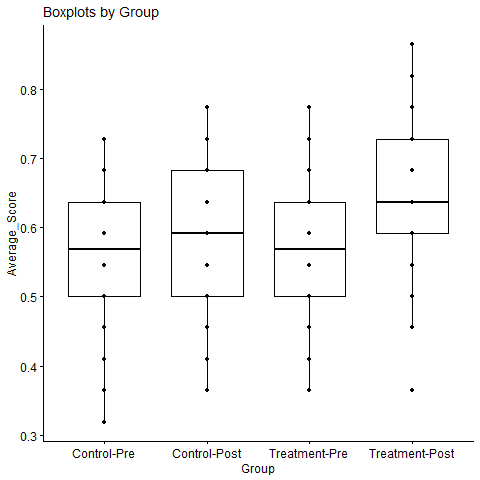One-way ANCOVA Boxplots

Second, the function runs scripts to check all assumptions to be satisfied and presents the results for users to confidently interpret the results of one-way ANCOVA. The first assumption checked and presented is linearity; the plot below can be used to check the linearity. If users are seeing a liner relationship between the covariate (i.e., pre-test scores for this analysis) and dependent variable (i.e., post-test scores for this analysis) for both treatment and control group in the plot, then they can say this assumption has been met.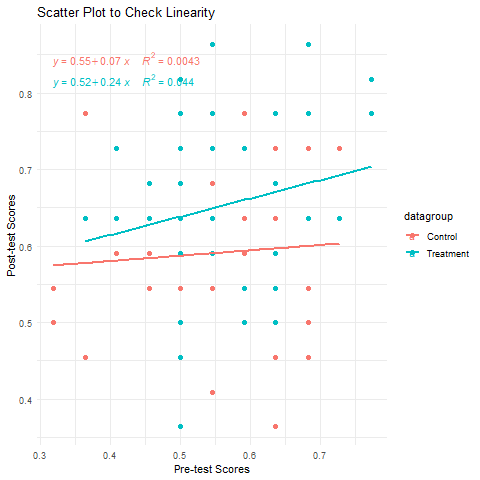One-way ANCOVA - Scatter Plot

#> ## Interpretation: if you are seeing a liner relationship between the covariate (i.e., pre-test scores for this analysis) and dependent variable (i.e., post-test scores for this analysis) for both treatment and control group in the plot, then you can say this assumption has been met or the data has not violated this assumption of linearity. If your relationships are not linear, you have violated this assumption, and an ANCOVA is not a siutable analysis. However, you might be able to coax your data to have a linear relationship by transforming the covariate, and still be able to run an ANCOVA.

Then, the assumption of normality of residuals is checked, and its result (with an interpretation) is presented, as shown below.

#> # Normality of Residuals:
#>
#>  Shapiro-Wilk normality test
#>
#> data:  norm.all.aov$residuals #> W = 0.98491, p-value = 0.3332 #> ## Interpretation: the assumption of normality by group has been met (p>0.05).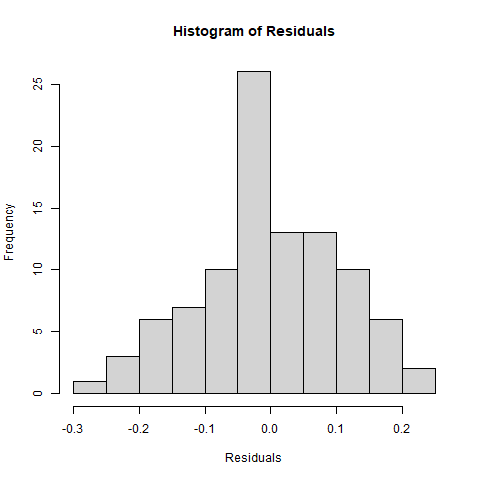One-way ANCOVA - Histogram Users need to visually examine the histogram (above) and normal Q-Q plot (below) as well to confirm the Shapiro-Wilk normality test result, especially when the sample size is large because the Shapiro-Wilk test becomes very sensitive to a minor deviation from normality at a larger sample size.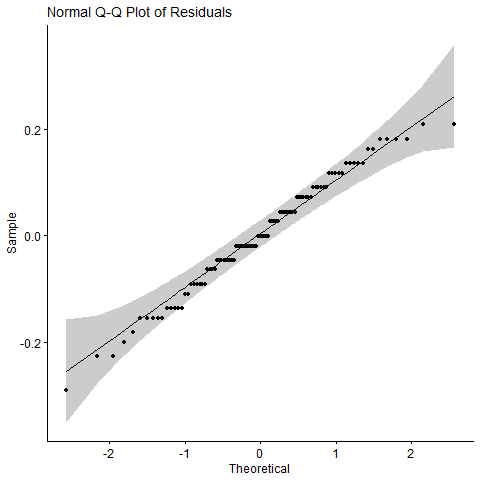One-way ANCOVA - Normal Q-Q Plot Then, the outputs present the result of checking homogeneity of variance. The function runs Levene Test and reports its result with an interpretation, as shown below. #> Levene's Test for Homogeneity of Variance (center = median) #> Df F value Pr(>F) #> group 1 0.0307 0.8613 #> 95 #> ## Interpretation: the assumption of equality of error variances has been met (p>0.05). Then, the function checks the data to see if any outlier exists and reports variables if an outlier is found, as shown below. #>  avg_score_post avg_score_pre datagroup .resid .cooksd #>  .std.resid #> <0 rows> (or 0-length row.names) #> # Outliers: No outlier has been found. Then, the last assumption of homogeneity of regression line slopes is checked, and its results (with an interpretation) are reported, as show below. #> #> Call: #> lm(formula = avg_score_post ~ avg_score_pre + datagroup + avg_score_pre:datagroup, #> data = full_data_binded) #> #> Residuals: #> Min 1Q Median 3Q Max #> -0.274339 -0.074246 0.007143 0.085445 0.214892 #> #> Coefficients: #> Estimate Std. Error t value Pr(>|t|) #> (Intercept) 0.55194 0.08774 6.290 1.02e-08 *** #> avg_score_pre 0.07016 0.15238 0.460 0.646 #> datagroupTreatment -0.03324 0.13148 -0.253 0.801 #> avg_score_pre:datagroupTreatment 0.16914 0.22750 0.743 0.459 #> --- #> Signif. codes: 0 '***' 0.001 '**' 0.01 '*' 0.05 '.' 0.1 ' ' 1 #> #> Residual standard error: 0.1088 on 93 degrees of freedom #> Multiple R-squared: 0.1014, Adjusted R-squared: 0.07246 #> F-statistic: 3.5 on 3 and 93 DF, p-value: 0.01856 #> ## Interpretation: there was homogeneity of regression slopes as the interaction term (i.e., datagroup:avg_score_pre) was not statistically significant (p>0.05). Third, upon presenting all results of checking the assumptions, the function generates the ANOVA table (type II tests) for users to examine the significance of the group variable. #> ANOVA Table (type II tests) #> #> Effect DFn DFd F p p<.05 ges #> 1 datagroup 1 94 8.148 0.005 * 0.080 #> 2 avg_score_pre 1 94 1.674 0.199 0.017 The function runs a post-hoc analysis to generate estimated (or adjusted) marginal means to compare between the groups and displays a summary statement of the outputs (see the results below). #> avg_score_pre datagroup emmean se df conf.low conf.high #> 1 0.5692887 Control 0.5920313 0.01505694 94 0.5621354 0.6219272 #> 2 0.5692887 Treatment 0.6551416 0.01618602 94 0.6230039 0.6872793 #> method #> 1 Emmeans test #> 2 Emmeans test #> --> A sample summary of the outputs/results above: The difference of post-test scores between the treatment and control groups turned out to be significant with pre-test scores being controlled: F(1,94)=8.148, p=0.005 (effect size=0.08). The adjusted marginal mean of post-test scores of the treatment group (0.66, SE=,0.02) was significantly different from that of the control group (0.59, SE=,0.02). ## One-way Repeated Measures ANOVA Function: “one_way_repeated_anova(treat_pre_csv_data, treat_post_csv_data, treat_post2_csv_data, m_cutoff = 0.15)” This function can be used when users collect data from the same students repeatedly at three different time points (e.g., pre-test, post-test, and second post-test) and want to examine the significance of the changes over time. The function automatically merges pre, post, and post2 data sets, runs the One-way repeated measures ANOVA with assumptions check, and then displays outputs all at once. An example without a file path: one_way_repeated_anova(treat_pre_csv_data = “data_treat_pre.csv”, treat_post_csv_data = “data_treat_post.csv”, treat_post2_csv_data = “data_treat_post2.csv”, m_cutoff = 0.1). If you provide only data file names, as shown in the function above, the folder where the file is saved should be set as the working directory. Alternatively, you can provide the path to the folder in the argument independent_samples Alternative example with a file path: one_way_repeated_anova(treat_pre_csv_data = “C:/Users/csong/documents/data_treat_pre.csv”, treat_post_csv_data = “C:/Users/csong/documents/data_treat_post.csv”, treat_post2_csv_data = “C:/Users/csong/documents/data_treat_post2.csv”, m_cutoff = 0.1). The ‘m_cutoff = 0.1’ in the function indicates removing students with 10 percent or more skipped answers (the default is 15 percent; m_cutoff = 0.15). This function automatically merges pre-post-post2 data sets, checks the assumptions of one-way ANCOVA, and then runs the main One-way ANCOVA all at once. The outputs from this function are as follows. First, the function presents descriptive statistics and boxplots of all three data sets, as shown below. #> # A tibble: 3 × 11 #> Time variable n min max median iqr mean sd se ci #> <fct> <fct> <dbl> <dbl> <dbl> <dbl> <dbl> <dbl> <dbl> <dbl> <dbl> #> 1 Pre Score 45 0.364 0.773 0.545 0.136 0.572 0.097 0.014 0.029 #> 2 Post1 Score 45 0.364 0.864 0.636 0.136 0.655 0.111 0.017 0.033 #> 3 Post2 Score 45 0.409 0.909 0.682 0.137 0.694 0.121 0.018 0.036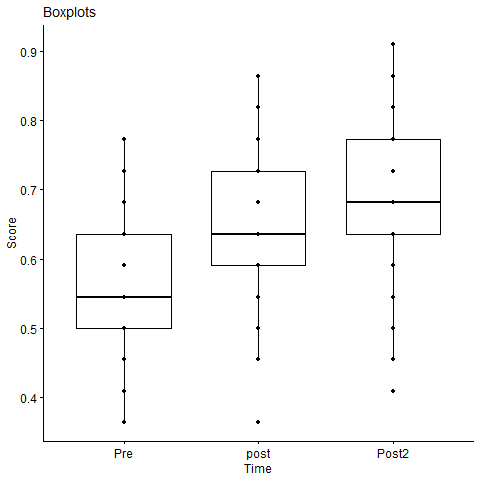One-way Repeated Measures ANOVA - Boxplots Second, the function runs scripts to check all assumptions to be satisfied and presents the results for users to confidently interpret the results of one-way repeated measures ANOVA. The first assumption checked is no outliers; the result is presented along with an interpretation, as shown below. #> ## Interpretation: No extreme outlier was identified in your data. Then, the assumption of normality of residuals is checked, and its result (with an interpretation) is presented, as shown below. #> #> Shapiro-Wilk normality test #> #> data: resid(res.aov) #> W = 0.98398, p-value = 0.1148 #> --> Interpretation: the residuals were normally distributed (p>0.05). Users need to visually examine the histogram and normal Q-Q plot as well to confirm the Shapiro-Wilk normality test result, especially when the sample size is large (e.g., greater than 50) because the Shapiro-Wilk test becomes very sensitive to a minor deviation from normality at a larger sample size.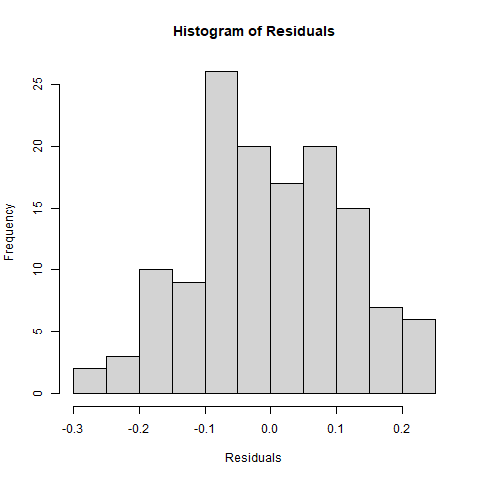One-way Repeated Measures ANOVA - Histogram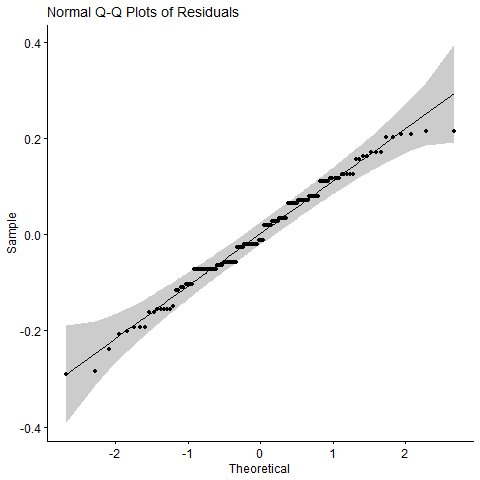One-way Repeated Measures ANOVA - Normal Q-Q Plot #> --> Interpretation: if all the points fall in the plots above approximately along the reference line, users can assume normality. Third, the function runs the one-way repeated measures ANOVA and presents its results, as shown below. The assumption of sphericity is checked as part of the computation of this ANOVA test. The Mauchly’s test has been internally run to assess the sphericity assumption. Then, the Greenhouse-Geisser sphericity correction has been automatically applied to factors violating the sphericity of assumption. #> ANOVA Table (type III tests) #> #> Effect DFn DFd F p p<.05 ges #> 1 Time 1.21 53.06 24.968 1.77e-06 * 0.18 #> --> Interpretation: The average test score at different time points of the intervention are statistically different: F(1.21 53.06)=24.968, p<0.001, eta2(g)=0.18. Then, if the result above turns out to be significant, then the function proceeds to conduct pairwise comparisons and present the results with an interpretation, as shown below. #> # A tibble: 3 × 10 #> .y. group1 group2 n1 n2 statistic df p p.adj p.adj.si…¹ #> * <chr> <chr> <chr> <int> <int> <dbl> <dbl> <dbl> <dbl> <chr> #> 1 Score Pre Post1 45 45 -4.29 44 0.0000963 0.000289 *** #> 2 Score Pre Post2 45 45 -5.52 44 0.0000017 0.0000051 **** #> 3 Score Post1 Post2 45 45 -4.70 44 0.000026 0.000078 **** #> # … with abbreviated variable name ¹​p.adj.signif #> --> Interpretation for 1: The average pre-test score (0.572) and the average post-test score (0.655) are significantly different. The average post-test score is significantly greater than the average pre-test score (p.adj<0.001). #> --> Interpretation for 2: The average post1-test score (0.655) and the average post2-test score (0.694) are significantly different. The average post2-test score is significantly greater than the average post-test score (p.adj<0.001). #> --> Interpretation for 3: The average pre-test score (0.572) and the average post2-test score (0.694) are significantly different. The average post2-test score is significantly greater than the average pre-test score (p.adj<0.001). Fourth, if the normality assumption is violated, the function continues to run the Friedman test, which is the non-parametric version of the parametric one-way repeated measures ANOVA. Although the one-way repeated measures ANOVA is known to be robust to a slight violation of the normality, users may want/need to refer to the result from the non-parametric Friedman test. (The following is just an illuatration of what users will see when the normality assumption is violated; that is, users won’t see this from testing the function with the provided sample data because it satisfies the assumption.) #> #> Friedman rank sum test #> #> data: avg_score_df #> Friedman chi-squared = 22.419, df = 2, p-value = 1.355e-05 #> --> Interpretation: the median test score is significantly different at the different time points during the intervention (p<0.001). Then, the result of pairwise comparisons with the non-normal data is presented with an interpretation. #> # A tibble: 3 × 9 #> .y. group1 group2 n1 n2 statistic p p.adj p.adj.signif #> * <chr> <chr> <chr> <int> <int> <dbl> <dbl> <dbl> <chr> #> 1 Score Pre Post1 45 45 146. 0.000233 0.000699 *** #> 2 Score Pre Post2 45 45 113 0.0000138 0.0000414 **** #> 3 Score Post1 Post2 45 45 0 0.0000919 0.000276 *** #> --> Interpretation for 1: the median pre-test score (0.545) and the median post-test score (0.636) are significantly different. The median post-test score is significantly greater than the median pre-test score (p.adj<0.001). #> --> Interpretation for 2: the median post-test score (0.636) and the median post2-test score (0.682) are significantly different. The median post2-test score is significantly greater than the median post-test score (p.adj<0.001). #> --> Interpretation for 3: the median pre-test score (0.545) and the median post2-test score (0.682) are significantly different. The median post2-test score is significantly greater than the median pre-test score (p.adj<0.001). ## Analysis of Demographic Group Differences Function: “demo_group_diff(score_csv_data, group_csv_data, m_cutoff = 0.15, group_name)” The ‘demogroupdiff()’ function requires users to type a data file name as shown in the sample code below. Users can input any of the data file names (e.g., “data_treat_pre.csv”, “data_treat_post.csv”) as they become available for the analysis of demographic group differences. An example without a file path: demo_group_diff(score_csv_data = “data_treat_pre.csv”, group_csv_data = “demographic_data.csv”, m_cutoff = 0.1, group_name = “grade”). If you provide only data file names, as shown in the function above, the folder where the file is saved should be set as the working directory. Alternatively, you can provide the path to the folder in the argument independent_samples Alternative example with a file path: demo_group_diff(score_csv_data = “C:/Users/csong/documents/data_treat_pre.csv”, group_csv_data = “C:/Users/csong/documents/demographic_data.csv”, m_cutoff = 0.1, group_name = “grade”). The ‘m_cutoff = 0.1’ in the function indicates removing students with 10 percent or more skipped answers (the default is 15 percent; m_cutoff = 0.15). This function automatically combines demographic variables to a dataset, and then runs the analysis of variance (ANOVA) with assumptions check to examine demographic sub-group differences for users all at once. The outputs/results from this function are as follows. In addition to the criteria to handle skipped answers (i.e., missing values), this function further asks users to choose a demographic variable to analyze. Users will see the demographic group variable names with a numeric code assigned (e.g., gender=1, grade=2). When asked “Enter the number assigned to the demographic variable name users want to analyze,” put the number in the R console and hit the ‘Enter’ key. The function runs all scripts at the back-end and presents the results automatically. The results from this function are as follows. First, the function presents a summary statistics for each demographic sub-group along with boxplots, as shown below. #> # A tibble: 4 × 5 #> group variable n mean sd #> <chr> <fct> <dbl> <dbl> <dbl> #> 1 Freshman average_score 11 0.562 0.091 #> 2 Junior average_score 14 0.594 0.103 #> 3 Senior average_score 10 0.532 0.113 #> 4 Sophomore average_score 15 0.573 0.087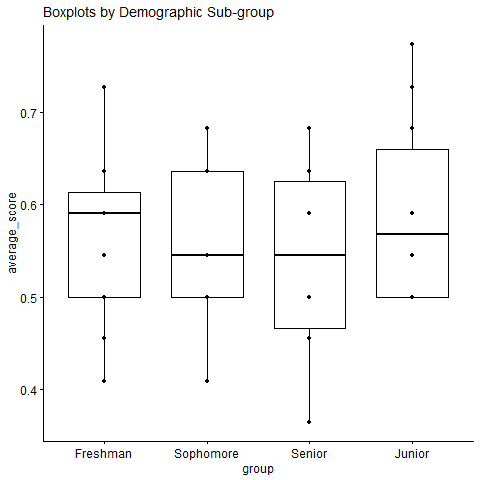Demographic Group Difference - Boxplots Second, the function continues to test the assumptions for the parametric one-way ANOVA. The first assumption checked is the normality of residuals; the Shapiro-Wilk test is performed, and its result is presented with an interpretation, as shown below. #> #> Shapiro-Wilk normality test #> #> data: resid(one_way) #> W = 0.96075, p-value = 0.09553 #> ## Interpretation: the assumption of normality by group has been met (p>0.05). If the sample size is greater than 50, it would be better refer to the normal Q-Q plot displayed in the ‘Plots’ panel to visually inspect the normality. This is because the Shapiro-Wilk test becomes very sensitive to a minor deviation from normality at a larger sample size. The plot is presented below with a guidance for interpretation.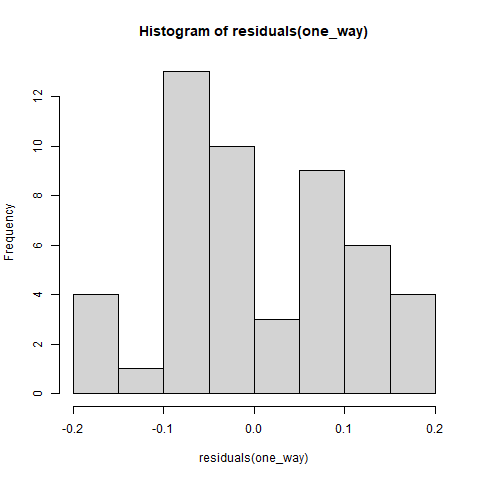Demographic Group Difference - Histogram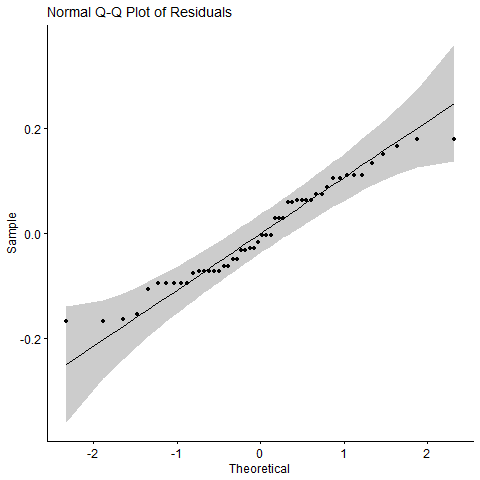Demographic Group Difference - Normal Q-Q Plot The second assumption checked is homogeneity of variance. The result is presented with an interpretation and a visual representation, as shown below. #> Levene's Test for Homogeneity of Variance (center = median) #> Df F value Pr(>F) #> group 3 0.371 0.7743 #> 46 #> ## Interpretation: the assumption of equality of variances has been met (p>0.05). Third, upon presenting the results of assumption checking, the function proceeds to run the one-way ANOVA with equal variances ‘assumed’ or ‘not assumed’ depending on the result of testing homegeneity of variance above. Then, the result with an interpretation is provided, as shown below. #> Results of One-way ANOVA: Group Difference(s) (Parametric: Equal variances assumed) #> Df Sum Sq Mean Sq F value Pr(>F) #> factor(group) 3 0.0233 0.007775 0.805 0.497 #> Residuals 46 0.4442 0.009657 #> --> Interpretation: the difference among the demographic sub-groups is not significant (P>0.05). #> Pairwide Comparisons (Equal variances assumed) Then, the function runs scripts to conduct pairwise comparisons and presents the result, as shown below. #> Pairwide Comparisons (Equal variances assumed) #> Tukey multiple comparisons of means #> 95% family-wise confidence level #> #> Fit: aov(formula = average_score ~ factor(group), data = data_original) #> #>$factor(group)
#>                           diff         lwr        upr     p adj
#> Junior-Freshman     0.03223377 -0.07330199 0.13776952 0.8474823
#> Senior-Freshman    -0.03000909 -0.14445579 0.08443761 0.8969661
#> Sophomore-Freshman  0.01069091 -0.09328546 0.11466728 0.9927074
#> Senior-Junior      -0.06224286 -0.17069336 0.04620765 0.4284339
#> Sophomore-Junior   -0.02154286 -0.11888016 0.07579445 0.9346245
#> Sophomore-Senior    0.04070000 -0.06623364 0.14763364 0.7418160

Fourth, in case the normality assumption is violated, the function continues to run the Kruskal-Wallis test, which is the non-parametric version of the parametric one-way ANOVA. Although ANOVA is known to be robust to a slight violation of the normality, users may want/need to refer to the result from the non-parametric Kruskal-Wallis test. The following is just an illuatration of what users will see when the normality assumption is violated; users won’t see this from testing the function with the provided sample data because it satisfies the assumption.

#> Results of Kruskal_Wallis Test (Non-parametric)
#>
#>  Kruskal-Wallis rank sum test
#>
#> data:  average_score by group
#> Kruskal-Wallis chi-squared = 1.412, df = 3, p-value = 0.7027
#> Interpretation: The difference among demographic sub-groups is not significant (p>0.05).
#>
#>  Pairwise comparisons using Wilcoxon rank sum test with continuity correction
#>
#> data:  data_original$average_score and data_original$group
#>
#>           Freshman Junior Senior
#> Junior    0.7      -      -
#> Senior    0.7      0.7    -
#> Sophomore 0.7      0.7    0.7
#>
#> P value adjustment method: BH